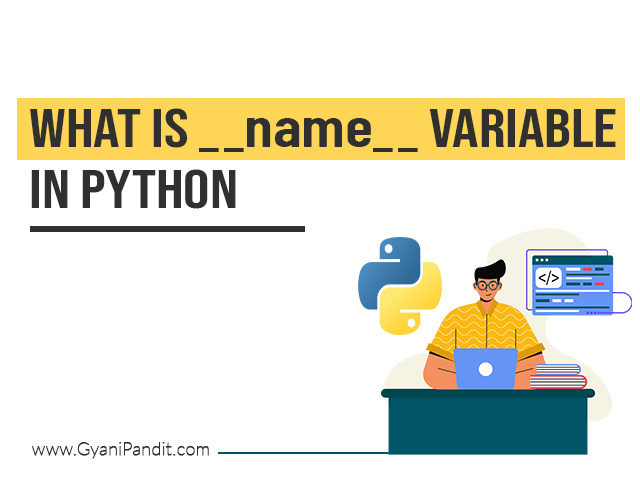# Python

## Check if all the elements in the list are the same in Python

In this article, we are going to address a simple problem in python, in which, we are going to have a list, and in that, we are going to check if all the elements in the list are the same. There can be multiple methods to achieve this task, and we are going to discuss …## Sum of all the digits in a given number Python program

In this article, we are going to understand and perform a simple and interesting python program, in which, we would have a number, and we need to calculate the sum of all the digits in the given number. Have a look at the below examples first, which give an idea about what are we required …## What is name variable in Python?

In many different python programs, you might have seen the statement if __name__ == “__main__”. So here in this article, we are going to understand a special variable __name__. We would see some different examples, through which, we can understand what is __name__ variable, and this would also give us some idea about the instruction …## What is an str method in Python?

In this article, we are going to understand the __str__ method in Python. This method can be said as the dunder str method, where the dunder means double underscores. These methods with double underscores are also called magic methods. So, the __str__ method returns the string representation of the object. Basically, the __str__ method is …